# Simplevis

#### 2021-06-04

``````library(dplyr)
library(simplevis)
library(palmerpenguins)
library(ggplot2)``````

### Purpose

simplevis is a package of wrapper functions that aim to make ggplot2 visualisation easier and quicker.

### Visualisation family types

`simplevis` supports the following families of visualisation type:

bar

``````plot_data <- storms %>%
group_by(year) %>%
summarise(wind = mean(wind))

gg_bar(plot_data, year, wind)``````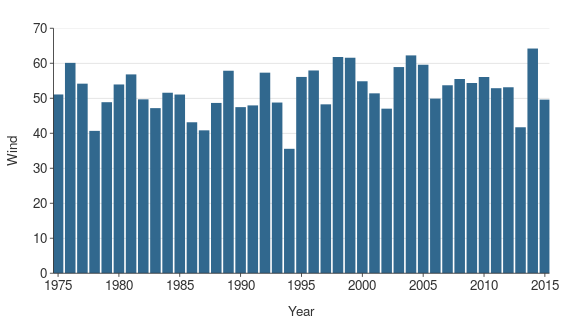point

``gg_point(iris, Sepal.Width, Sepal.Length)``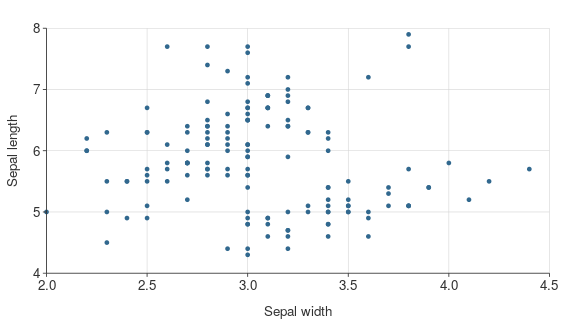line

``````plot_data <- storms %>%
group_by(year) %>%
summarise(wind = mean(wind))

gg_line(plot_data, year, wind)``````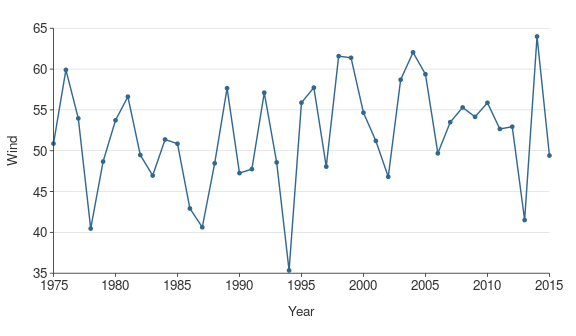• boxplot
``gg_boxplot(storms, year, wind)``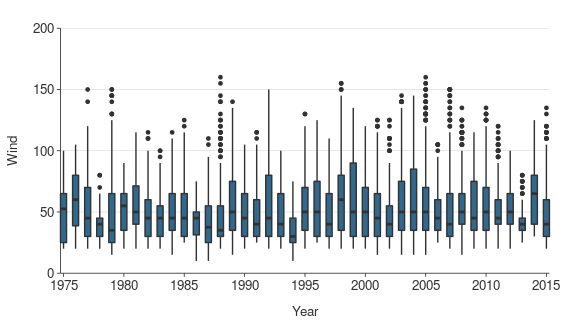hbar (i.e horizontal bar)

``````plot_data <- ggplot2::diamonds %>%
group_by(cut) %>%
summarise(price = mean(price))

gg_hbar(plot_data, price, cut)``````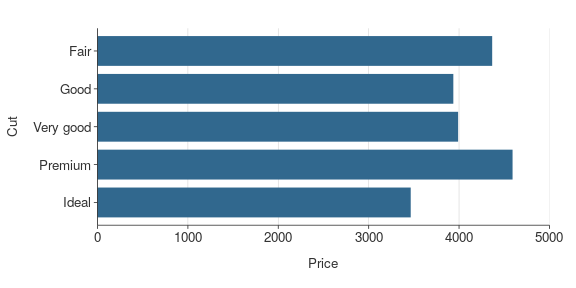• sf (short for simple features map)
``gg_sf(example_sf_point, borders = nz)``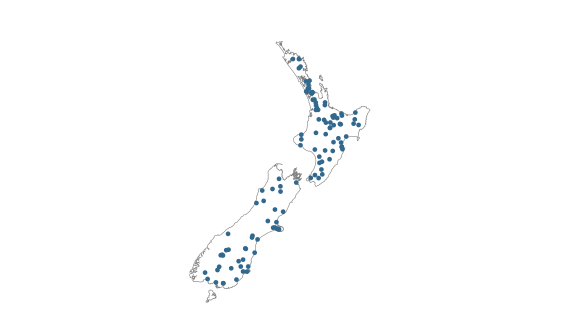### Colouring, facetting, neither or both

Each visualisation family generally has 4 functions.

The function name specifies whether or not a visualisation is to be coloured by a variable (`*_col()`), facetted by a variable (`*_facet()`), or neither (`*()`) or both of these (`*_col_facet()`).

Colouring by a variable means that different values of a selected variable are to have different colours. Facetting means that different values of a selected variable are to have their facet.

A `*()` function such `gg_point()` requires only a dataset, an x variable and a y variable.

``gg_point(penguins, bill_length_mm, body_mass_g)``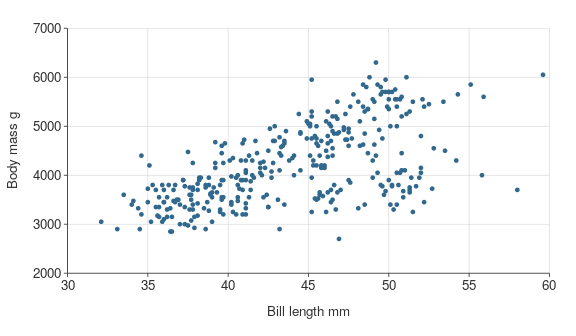A `*_col()` function such `gg_point_col()` requires only a dataset, an x variable, a y variable, and a colour variable.

``gg_point_col(penguins, bill_length_mm, body_mass_g, sex)``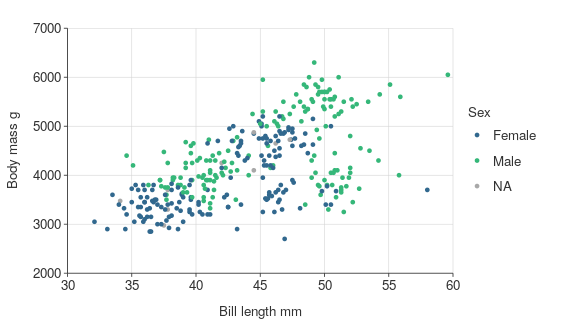A `*_facet()` function such `gg_point_facet()` requires only a dataset, an x variable, a y variable, and a facet variable.

``gg_point_facet(penguins, bill_length_mm, body_mass_g, species)``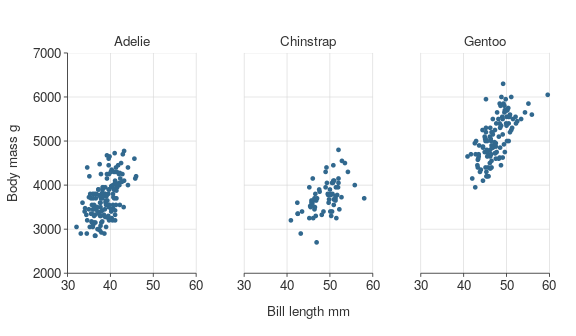A `*_col_facet()` function such `gg_point_col_facet()` requires only a dataset, an x variable, a y variable, a colour variable, and a facet variable.

``gg_point_col_facet(penguins, bill_length_mm, body_mass_g, sex, species)``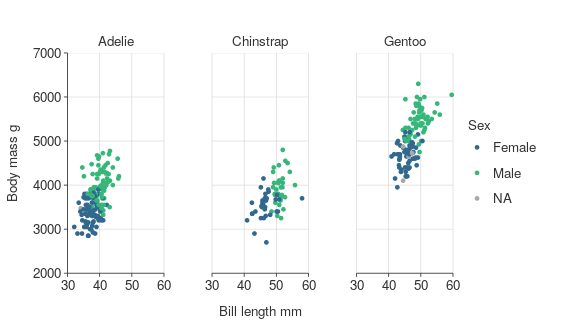Data is generally plotted with a stat of `identity`, which means data is plotted as is. Only for boxplot, there is a different default stat of boxplot, which means data will be transformed to boxplot statistics.

`_sf` functions for maps differ slightly, which is discussed further below.

### Titles

Customise titles with `title`, `subtitle`, `x_title`, `y_title` and `caption` arguments.

``````gg_point_col(penguins, bill_length_mm, body_mass_g, species,
title = "Adult penguin mass by bill length and species",
subtitle = "Palmer station, Antarctica",
x_title = "Bill length (mm)",
y_title = "Body mass (g)",
col_title = "Penguin species",
caption = "Source: Gorman KB, Williams TD, Fraser WR (2014)")``````